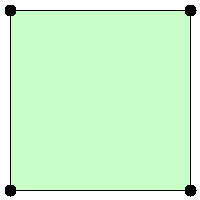## 469. Convex Polygon

Given a list of points that form a polygon when joined sequentially, find if this polygon is convex (Convex polygon definition).

Note:

1. There are at least 3 and at most 10,000 points.
2. Coordinates are in the range -10,000 to 10,000.
3. You may assume the polygon formed by given points is always a simple polygon (Simple polygon definition). In other words, we ensure that exactly two edges intersect at each vertex, and that edges otherwise don't intersect each other.

Example 1:

```[[0,0],[0,1],[1,1],[1,0]]

Explanation:```

Example 2:

```[[0,0],[0,10],[10,10],[10,0],[5,5]]

Explanation:```

## Rust Solution

``````struct Solution;

impl Solution {
fn is_convex(points: Vec<Vec<i32>>) -> bool {
let n = points.len();
let mut positive = 0;
let mut negative = 0;
for i in 0..n {
let sign = Self::cross(&points[i], &points[(i + 1) % n], &points[(i + 2) % n]);
if sign >= 0 {
positive += 1;
}
if sign <= 0 {
negative += 1;
}
}
positive == n || negative == n
}

fn cross(a: &[i32], b: &[i32], c: &[i32]) -> i32 {
(b - a) * (c - b) - (b - a) * (c - b)
}
}

#[test]
fn test() {
let points = vec_vec_i32![[0, 0], [0, 1], [1, 1], [1, 0]];
let res = true;
assert_eq!(Solution::is_convex(points), res);
let points = vec_vec_i32![[0, 0], [0, 10], [10, 10], [10, 0], [5, 5]];
let res = false;
assert_eq!(Solution::is_convex(points), res);
}
``````

Having problems with this solution? Click here to submit an issue on github.